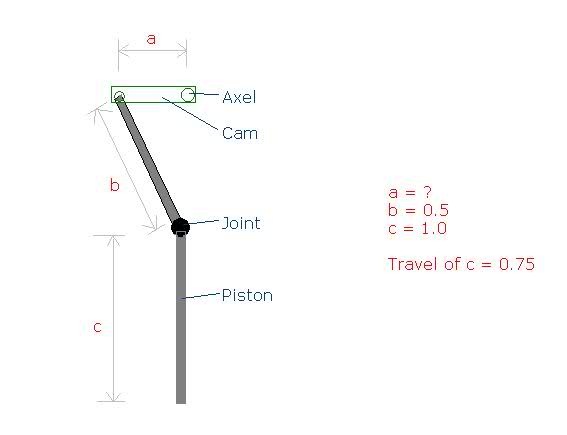# Working out Cam dimensions

Hi,

Im trying to work out the dimensions of the offset on a cam for a project. I know the travel of rod c, as well as the length of the connecting rod b, but im not sure what the lenth of dimension a should be. I know this is probably simple maths (just pythagoras?) but I need to make sure I get the full travel out of rod c with full 360 degree rotation on the axel.

It would normally be very simple (a = 0.5 x travel of rod c), but it is the fact that rod b is allowed to pivot around the joint to rod c makes it a bit more confusing.#### Attachments

HallsofIvy
Homework Helper
c will be highest when a is pointing directly upward. At that position, the distance from the Axle to the tip of c is b+ c- a= 1.5- a. c will be lowest when a is pointing directly downward. At that position, the distance from the Axle to the tip of c is a+ b+ c= a+ 1.5. The distance between those, the "travel" is a+1.5- (1.5- a)= 2a. You want a equal to 1/2 the travel just as you said. The fact that b pivots changes the speed with which c travels but not the distance.

As long as the axel is in line with the piston, the cam "a" needs to be half the length of the travel desired at the piston. Therefore, since the cylinder travel is 3/4 units, the cam should be 3/8 units.

The Pythagorean theorem comes into play when the axel is NOT in line with the cylinder.

c will be highest when a is pointing directly upward.\

... unless the axle is not in line with the cylinder. In that case (in fact, in all cases), cylinder "C" will be highest when the pivot point connecting cam "A" and rod "B" is in a position such that the axle is directly between that pivot point and the joint between rod "B" and cylinder "C"